# Using Matlab from C# application.

While writing an application for my Masters degree diploma, I wrote a simple application that was using COM Matlab server. I have found it hard to use it mainly due to lack of documentation, which is really basic with only few code examples for C#. I guess writing programs that use Matlab for calculating is not encouraged by MathWorks, you would became competition that way 🙂 Nonetheless, I accomplished why I was required to do, so I decided to share this with the rest of the world.

Important: I was using R2010a version of Matlab. I realize that there is a newer version. But I had only this version at my disposal. Since interface for communicating with Matlab server is dependent on installed Matlab version and registry entries, it may have been different from yours. But I suspect not too much. I also tried with 7.1 and (if I remember correctly) it required only to swap reference in Visual Studio. But again… it was only a test so there might be other problems that I am not aware of.

Let’s start with a simple console application. Let’s call it `MatlabTest`. First, we will add DLL reference with COM interface. Click RMB on project and choose [Add Reference] option. In new window, click COM tab. In search text box, write ‘Matlab’. Then choose “Matlab Application (Version 7.10) Type Library”.

You should get a new reference like below: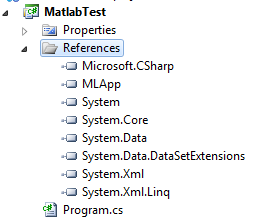Great. Now we should test if it is working. In order to use it, we should create our Matlab server from C# application. To do that, we can add code to our main program:

```var activationContext = Type.GetTypeFromProgID("matlab.application.single");
var matlab = (MLApp.MLApp)Activator.CreateInstance(activationContext);
Console.WriteLine(matlab.Execute("1+2"));

This code will create a single Matlab COM server through `Activator` class. Program Id `"matlab.application.single"` means single Matlab COM server for our application. When it will try to create another Matlab, it will just return a reference to the same object. Contrary to that, we could use `"matlab.application"` which will create another instance of Matlab anytime `Activator.CreateIntance` method will be executed. In more complex applications or for web applications or other programs which run for a long time, it may create big memory leaks since 1 instance costs around 220 Mb (on 64 bit Windows 7 and Matlab R2010a).

After creating Matlab program, execute a simple sum of 2 integers, just for testing communication – we don’t need anything more sophisticated. It should return also a simple `string` in console: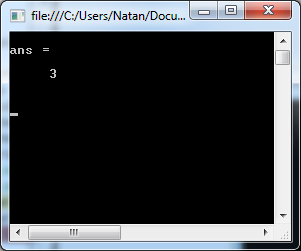It’s really simple and more importantly, it works! 🙂

This way, we can send and receive `string` messages with MATLAB only. It’s not very useful. Also, there is one way to find out if our statement had errors. It will have `error` string in response.

Let’s try to run something like this: `1*`, which will result in error: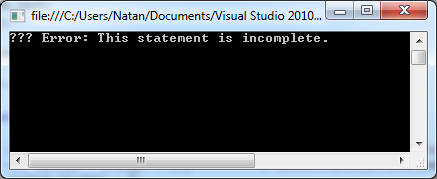So to check our command had errors, we have to check if output `string` has `??? Error` in it.

To send some parameters along with our command, we have to use one of `Put*`* methods. Best is one called `PutWorkspaceData`. It takes three parameters. First is the desired name of our new Matlab variable. Two others are much more tricky. To set variable correctly (so you could reference it in command), you must use global workspace. But it is called? This one took much more time than I would want. It is not mentioned in the documentation of this method. If I remember it right, I found it in some code example and it should be only `base`. In the end, I created in my application another method that encapsulated `PutWorkspaceData` and forgot about it 🙂 The third parameter is the value of our variable. It should be simple. Let’s change our code to:

```matlab.PutWorkspaceData("a", "base", 2);
Console.WriteLine(matlab.Execute("a*a"));```

The result will be as shown below: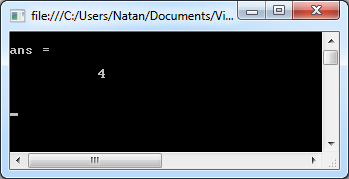But it is just `int`. What about more complicated structures? How about multiplication of two vectors? Matlab is using .NET type `double` to send and receive information with our application. Again, I did not find it anywhere in the documentation, but rather reverse engineered this from data returned from Matlab. So let us try to send 2 arrays of doubles. and multiply them in Matlab. First will be named `a` and second `b`. Matlab command will be `"a'*b"`. Transposition will give us a nice matrix instead for a single number.

```matlab.PutWorkspaceData("a", "base", new[]{2d,3d,1d});
matlab.PutWorkspaceData("b", "base", new[]{4d,3d,2d});
Console.WriteLine(matlab.Execute("a'*b"));```

And in return we will get: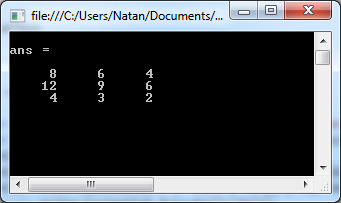Next step will be to return this output to our console app. To do that, we can use `GetWorkspaceData` that works similar to `PutWorkspaceData` or… we can use `GetVariable` method. This one returns `dynamic` so our application needs to run on .NET 4. It takes two parameters – name of variable we want to return from Matlab and again name of workspace. You really should save this `string` as `const` somewhere 🙂 Change our code to:

```matlab.PutWorkspaceData("a", "base", new[]{2d,3d,1d});
matlab.PutWorkspaceData("b", "base", new[]{4d,3d,2d});
Console.WriteLine(matlab.Execute("c=a'*b"));
var c = matlab.GetVariable("c", "base");```

After that, in our console app, we will have variable `c` and it will be a two dimensional array of `double`s. To show values of this array in console, we can just iterate it with a simple `foreach` loop. But instead of that, we will iterate two of the dimensions. This will give us information about values and dimensions of this matrix.

```for (var i = 0; i < c.GetLength(0); i++)
{
for (var j = 0; j < c.GetLength(1); j++)
Console.Write(c.GetValue(i, j) + " ");
Console.WriteLine();
}```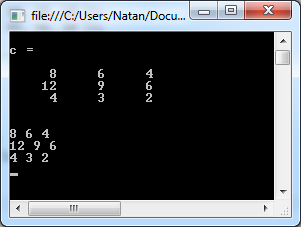Ok.That was matrix. How about vectors? Luckily, vectors are for Matlab just a special case of matrix so it will spit out two dimensional array also. More complex is the case of empty matrix. No, it is not `null`. It would be too easy. Instead, it is a special type `Missing`. I guess it has some logic behind. `null` would indicate that variable will have no value at all or this is not defined. But we have an empty matrix, so this is not defined nor is this a lack of value. So why not just array with zero values in it? No idea.

Let’s try to run code like below to test it:

```Console.WriteLine(matlab.Execute("c=a'*b"));
Console.WriteLine(matlab.Execute("d=[]"));
var c = matlab.GetVariable("c", "base");
var d = matlab.GetVariable("d", "base");
for (var i = 0; i < d.GetLength(0); i++)
{
for (var j = 0; j < d.GetLength(1); j++)
Console.Write(d.GetValue(i, j) + " ");
Console.WriteLine();
}```

Running those commands in Matlab will work just fine. Instead of that, error will be thrown on first `for` loop: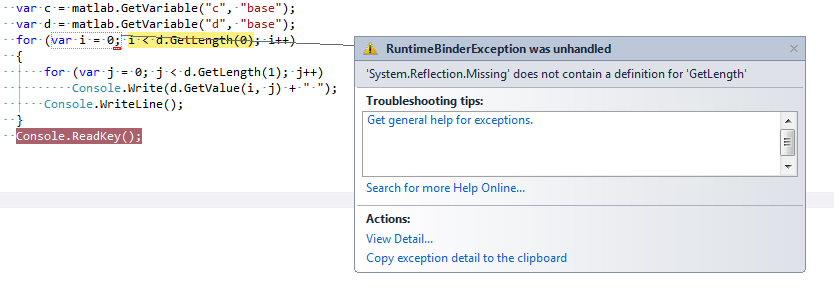Not very nice. To prevent this error, the application will have to check if dynamic type returned from matlab is in fact empty (`Missing`). Not very clean it is better to wrap this in other method that will perform this check for us whenever we want to get our data from Matlab. In my project, I ended up writing few methods for returning vectors, matrices, numbers, strings, etc. `Vector` one looked like this:

```public static Vector GetVector(this MLApp.MLApp matlabComObject, string variabla_name)
{
var dynamicVariable = matlabComObject.GetBaseWorkSpaceVariable(variabla_name);
var dataList = new Vector();

if (TypeChecker.IsVector(dynamicVariable))
{
dataList = Vector.DynamicToVector(dynamicVariable);
}
else if (TypeChecker.IsNumber(dynamicVariable))
{
}
else if (TypeChecker.IsEmptyMatrix(dynamicVariable))
{
//do nothing empty vector or matrix ([0x0] or [1x0])
}
else throw new Exception(string.Format(
"Type of dynamic variable ({0}) is not supported!", dynamicVariable.GetType()));
return dataList;
}```

`TypeCheker` checks for type of `dynamic`. It’s pretty straightforward. For our `Missing` type, it’s just one line:

```public static bool IsEmptyMatrix(object dynamicVariable)
{ return dynamicVariable.GetType() == typeof(Missing); }```

After checking type of `dynamic`, we can just cast it to another type to take advantage of `static` properties of language. Why use `dynamic` at all? First, I think that `ref` and `out` method parameters are messy and second: I prefer:

`var d = matlab.GetVariable("d", "base");`

from:

```object e;
matlab.GetWorkspaceData("d", "base", out e);```

It is only 1 line. And since object you have to create first and then cast it also, don’t bother and just use `dynamic`. But I guess it is just what you will like better.

`string`s are much more friendly and there are just `string`. Or `null` 🙂

Now we know how to put and get data from Matlab. How to execute commands and check them for errors.

If you executed any of these examples, you probably took notice of a very simple Matlab window which had opened during the life of your console app. If you terminate it by disabling debugging or it will close due to error, Matlab window will remain opened. If not, it will close nicely with console window. But still, it is a huge memory leak risk if not maintained properly. To that, I recommend creating some special class that will create an instance or instances of Matlab. It should track one of the created instances and in case anything bad happens (but dynamic variable cast is probability :), it will close all instances prior to application exit. Tracking should be employed through `WeakReference` class, so whenever Garbage Collector would want to destroy Matlab instance, it should not be stopped by our tracking class. To destroy COM instance, we can use method `Marshal.FinalReleaseComObject`. So with weak reference code for that will look like this:

```public static void Release(WeakReference instance)
{
if (instance.IsAlive)
{
Marshal.FinalReleaseComObject(instance.Target);
}
}```

Method `Quit` of matlab server instance does not close it immediately and the code above will.

If you want to hide that popup Matlab window, you can set this in its instance:

`matlab.Visible = 0;`

This way, it will fade away and run as service.

This is the most basic information about Matlab in C#, but it will get you started. So happy coding! 🙂

## 9 Replies to “Using Matlab from C# application.”

1.sadsad says:

Do I need to have matlab installed on computer to use this example program if you send me .exe file?

2.natan says:

Thanks all for nice comments! 🙂
@sadsad: Of course you need Matlab installed. This particular piece of code is using COM interface which is registered during Matlab installation.

3.Gerardo Macias says:

hello. im interested in your code but i have a few doubts. first of all do i need to save a Matlab file? if so with which name i need to save it?

i have problems running this

var activationContext = Type.GetTypeFromProgID("matlab.application.single");
var matlab = (MLApp.MLApp)Activator.CreateInstance(activationContext);
Console.WriteLine(matlab.Execute("1+2"));

it sends me an error with Xamlparseexception

4.natan says:

[quote]hello. im interested in your code but i have a few doubts. first of all do i need to save a Matlab file? if so with which name i need to save it?
i have problems running this [/quote]
It is not about matlab files. It is C# code written in .cs files in Visual Studio.
Also I do not understand how my C# code could give you XamlParseException. This has nothing to do with Xaml. Please follow my explanation and create console application. This example was tested thoroughly and work with Matlab R2010a.

5.vijay says:

Hi, is it possible that after invoking matlab function using feval from C# to get the figure into C# app. assuming following.
1. i can't modify matlab script and this script is not saving the graph whatsoever.
2. i need to make a report and so catch this figure generated by matlab from my c# app.

6.Dushyant says:

Hi,
How can i invoke this code to plot dynamic data? I have written some code, I am able to display sum etc.. but not able to plot the data. Any advice will be helpful

7.natan says:

>Hi,
>How can i invoke this code to plot dynamic data?

You need to write code to read plot data from Matlab export it to .NET app and show it via some graphic library.

8.Mark says:

nice one!

can you post the classes vector, matrix, string etc as well?

9.natan says:

>can you post the classes vector, matrix, string etc as well?
It is old article I am not sure if I had this code stashed somewhere. I need to look for it. Send me and email if you still need it – I will look for it